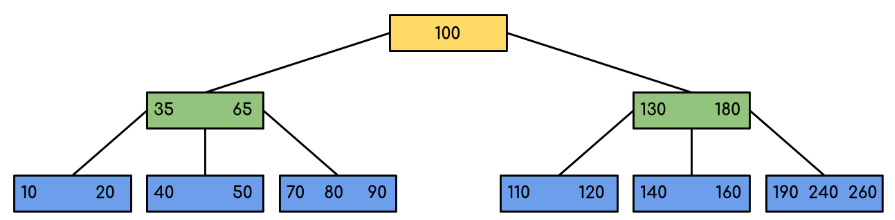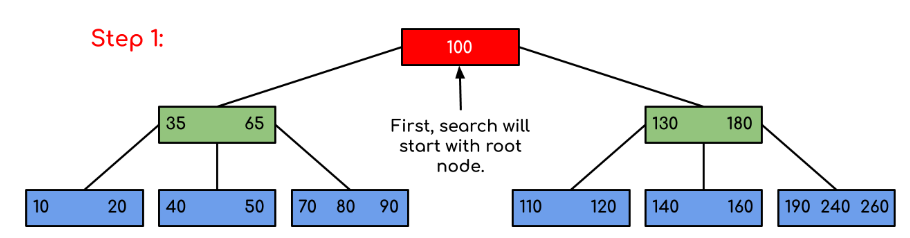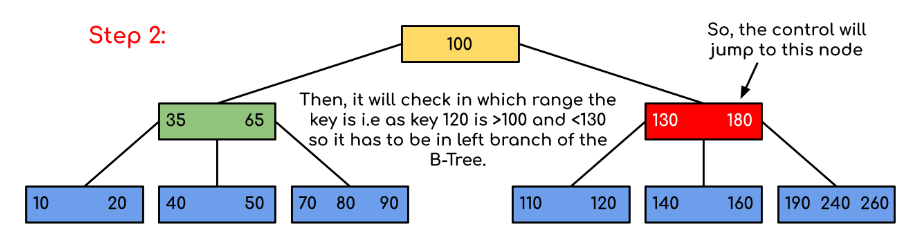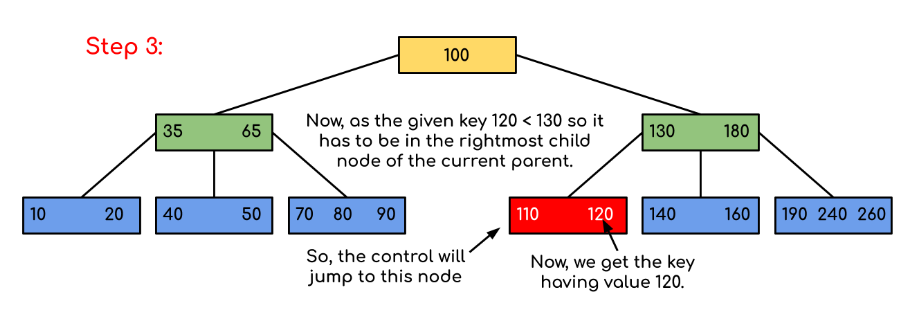Open In App

# Introduction of B-Tree

The limitations of traditional binary search trees can be frustrating. Meet the B-Tree, the multi-talented data structure that can handle massive amounts of data with ease. When it comes to storing and searching large amounts of data, traditional binary search trees can become impractical due to their poor performance and high memory usage. B-Trees, also known as B-Tree or Balanced Tree, are a type of self-balancing tree that was specifically designed to overcome these limitations.

Unlike traditional binary search trees, B-Trees are characterized by the large number of keys that they can store in a single node, which is why they are also known as “large key” trees. Each node in a B-Tree can contain multiple keys, which allows the tree to have a larger branching factor and thus a shallower height. This shallow height leads to less disk I/O, which results in faster search and insertion operations. B-Trees are particularly well suited for storage systems that have slow, bulky data access such as hard drives, flash memory, and CD-ROMs.

B-Trees maintain balance by ensuring that each node has a minimum number of keys, so the tree is always balanced. This balance guarantees that the time complexity for operations such as insertion, deletion, and searching is always O(log n), regardless of the initial shape of the tree.

## Time Complexity of B-Tree:

Sr. No.AlgorithmTime Complexity
1.SearchO(log n)
2.InsertO(log n)
3.DeleteO(log n)

Note: “n” is the total number of elements in the B-tree

## Properties of B-Tree:

• All leaves are at the same level.
• B-Tree is defined by the term minimum degree ‘t‘. The value of ‘t‘ depends upon disk block size.
• Every node except the root must contain at most t-1 keys. The root may contain a minimum of 1 key.
• All nodes (including root) may contain at most (2*t – 1) keys.
• Number of children of a node is equal to the number of keys in it plus 1.
• All keys of a node are sorted in increasing order. The child between two keys k1 and k2 contains all keys in the range from k1 and k2.
• B-Tree grows and shrinks from the root which is unlike Binary Search Tree. Binary Search Trees grow downward and also shrink from downward.
• Like other balanced Binary Search Trees, the time complexity to search, insert and delete is O(log n).
• Insertion of a Node in B-Tree happens only at Leaf Node.

Following is an example of a B-Tree of minimum order 5
Note: that in practical B-Trees, the value of the minimum order is much more than 5.We can see in the above diagram that all the leaf nodes are at the same level and all non-leafs have no empty sub-tree and have keys one less than the number of their children.

• The minimum height of the B-Tree that can exist with n number of nodes and m is the maximum number of children of a node can have is:• The maximum height of the B-Tree that can exist with n number of nodes and t is the minimum number of children that a non-root node can have is:and## Traversal in B-Tree:

Traversal is also similar to Inorder traversal of Binary Tree. We start from the leftmost child, recursively print the leftmost child, then repeat the same process for the remaining children and keys. In the end, recursively print the rightmost child.

## Search Operation in B-Tree:

Search is similar to the search in Binary Search Tree. Let the key to be searched is k.

• Start from the root and recursively traverse down.
• For every visited non-leaf node,
• If the node has the key, we simply return the node.
• Otherwise, we recur down to the appropriate child (The child which is just before the first greater key) of the node.
• If we reach a leaf node and don’t find k in the leaf node, then return NULL.

Searching a B-Tree is similar to searching a binary tree. The algorithm is similar and goes with recursion. At each level, the search is optimized as if the key value is not present in the range of the parent then the key is present in another branch. As these values limit the search they are also known as limiting values or separation values. If we reach a leaf node and don’t find the desired key then it will display NULL.

## C++

 struct Node {    int n;    int key[MAX_KEYS];    Node* child[MAX_CHILDREN];    bool leaf;}; Node* BtreeSearch(Node* x, int k) {    int i = 0;    while (i < x->n && k >= x->key[i]) {        i++;    }    if (i < x->n && k == x->key[i]) {        return x;    }    if (x->leaf) {        return nullptr;    }    return BtreeSearch(x->child[i], k);}

## C

 BtreeSearch(x, k)    i = 1         // n[x] means number of keys in x node    while i ≤ n[x] and k ≥ keyi[x]        do i = i + 1    if i  n[x] and k = keyi[x]        then return (x, i)      if leaf [x]        then return NIL    else        return BtreeSearch(ci[x], k)

## Java

 class Node {    int n;    int[] key = new int[MAX_KEYS];    Node[] child = new Node[MAX_CHILDREN];    boolean leaf;} Node BtreeSearch(Node x, int k) {    int i = 0;    while (i < x.n && k >= x.key[i]) {        i++;    }    if (i < x.n && k == x.key[i]) {        return x;    }    if (x.leaf) {        return null;    }    return BtreeSearch(x.child[i], k);}

## Python3

 class Node:    def __init__(self):        self.n = 0        self.key =  * MAX_KEYS        self.child = [None] * MAX_CHILDREN        self.leaf = True def BtreeSearch(x, k):    i = 0    while i < x.n and k >= x.key[i]:        i += 1    if i < x.n and k == x.key[i]:        return x    if x.leaf:        return None    return BtreeSearch(x.child[i], k)

## C#

 class Node {    public int n;    public int[] key = new int[MAX_KEYS];    public Node[] child = new Node[MAX_CHILDREN];    public bool leaf;} Node BtreeSearch(Node x, int k) {    int i = 0;    while (i < x.n && k >= x.key[i]) {        i++;    }    if (i < x.n && k == x.key[i]) {        return x;    }    if (x.leaf) {        return null;    }    return BtreeSearch(x.child[i], k);}

## Javascript

 // Define a Node class with properties n, key, child, and leafclass Node {    constructor() {        this.n = 0;        this.key = new Array(MAX_KEYS);        this.child = new Array(MAX_CHILDREN);        this.leaf = false;    }} // Define a function BtreeSearch that takes in a Node object x and an integer kfunction BtreeSearch(x, k) {    let i = 0;    while (i < x.n && k >= x.key[i]) {        i++;    }    if (i < x.n && k == x.key[i]) {        return x;    }    if (x.leaf) {        return null;    }    return BtreeSearch(x.child[i], k);}

Examples:

Input: Search 120 in the given B-Tree.Solution:In this example, we can see that our search was reduced by just limiting the chances where the key containing the value could be present. Similarly if within the above example we’ve to look for 180, then the control will stop at step 2 because the program will find that the key 180 is present within the current node. And similarly, if it’s to seek out 90 then as 90 < 100 so it’ll go to the left subtree automatically, and therefore the control flow will go similarly as shown within the above example.

Below is the implementation of the above approach:

## C++

 // C++ implementation of search() and traverse() methods#include using namespace std; // A BTree nodeclass BTreeNode {    int* keys; // An array of keys    int t; // Minimum degree (defines the range for number           // of keys)    BTreeNode** C; // An array of child pointers    int n; // Current number of keys    bool leaf; // Is true when node is leaf. Otherwise falsepublic:    BTreeNode(int _t, bool _leaf); // Constructor     // A function to traverse all nodes in a subtree rooted    // with this node    void traverse();     // A function to search a key in the subtree rooted with    // this node.    BTreeNode*    search(int k); // returns NULL if k is not present.     // Make the BTree friend of this so that we can access    // private members of this class in BTree functions    friend class BTree;}; // A BTreeclass BTree {    BTreeNode* root; // Pointer to root node    int t; // Minimum degreepublic:    // Constructor (Initializes tree as empty)    BTree(int _t)    {        root = NULL;        t = _t;    }     // function to traverse the tree    void traverse()    {        if (root != NULL)            root->traverse();    }     // function to search a key in this tree    BTreeNode* search(int k)    {        return (root == NULL) ? NULL : root->search(k);    }}; // Constructor for BTreeNode classBTreeNode::BTreeNode(int _t, bool _leaf){    // Copy the given minimum degree and leaf property    t = _t;    leaf = _leaf;     // Allocate memory for maximum number of possible keys    // and child pointers    keys = new int[2 * t - 1];    C = new BTreeNode*[2 * t];     // Initialize the number of keys as 0    n = 0;} // Function to traverse all nodes in a subtree rooted with// this nodevoid BTreeNode::traverse(){    // There are n keys and n+1 children, traverse through n    // keys and first n children    int i;    for (i = 0; i < n; i++) {        // If this is not leaf, then before printing key[i],        // traverse the subtree rooted with child C[i].        if (leaf == false)            C[i]->traverse();        cout << " " << keys[i];    }     // Print the subtree rooted with last child    if (leaf == false)        C[i]->traverse();} // Function to search key k in subtree rooted with this nodeBTreeNode* BTreeNode::search(int k){    // Find the first key greater than or equal to k    int i = 0;    while (i < n && k > keys[i])        i++;     // If the found key is equal to k, return this node    if (keys[i] == k)        return this;     // If the key is not found here and this is a leaf node    if (leaf == true)        return NULL;     // Go to the appropriate child    return C[i]->search(k);}

## Java

 // Java program to illustrate the sum of two numbers // A BTreeclass Btree {    public BTreeNode root; // Pointer to root node    public int t; // Minimum degree     // Constructor (Initializes tree as empty)    Btree(int t)    {        this.root = null;        this.t = t;    }     // function to traverse the tree    public void traverse()    {        if (this.root != null)            this.root.traverse();        System.out.println();    }     // function to search a key in this tree    public BTreeNode search(int k)    {        if (this.root == null)            return null;        else            return this.root.search(k);    }} // A BTree nodeclass BTreeNode {    int[] keys; // An array of keys    int t; // Minimum degree (defines the range for number           // of keys)    BTreeNode[] C; // An array of child pointers    int n; // Current number of keys    boolean        leaf; // Is true when node is leaf. Otherwise false     // Constructor    BTreeNode(int t, boolean leaf)    {        this.t = t;        this.leaf = leaf;        this.keys = new int[2 * t - 1];        this.C = new BTreeNode[2 * t];        this.n = 0;    }     // A function to traverse all nodes in a subtree rooted    // with this node    public void traverse()    {         // There are n keys and n+1 children, traverse        // through n keys and first n children        int i = 0;        for (i = 0; i < this.n; i++) {             // If this is not leaf, then before printing            // key[i], traverse the subtree rooted with            // child C[i].            if (this.leaf == false) {                C[i].traverse();            }            System.out.print(keys[i] + " ");        }         // Print the subtree rooted with last child        if (leaf == false)            C[i].traverse();    }     // A function to search a key in the subtree rooted with    // this node.    BTreeNode search(int k)    { // returns NULL if k is not present.         // Find the first key greater than or equal to k        int i = 0;        while (i < n && k > keys[i])            i++;         // If the found key is equal to k, return this node        if (keys[i] == k)            return this;         // If the key is not found here and this is a leaf        // node        if (leaf == true)            return null;         // Go to the appropriate child        return C[i].search(k);    }}

## Python3

 # Create a nodeclass BTreeNode:  def __init__(self, leaf=False):    self.leaf = leaf    self.keys = []    self.child = []  # Treeclass BTree:  def __init__(self, t):    self.root = BTreeNode(True)    self.t = t     # Insert node  def insert(self, k):    root = self.root    if len(root.keys) == (2 * self.t) - 1:      temp = BTreeNode()      self.root = temp      temp.child.insert(0, root)      self.split_child(temp, 0)      self.insert_non_full(temp, k)    else:      self.insert_non_full(root, k)     # Insert nonfull  def insert_non_full(self, x, k):    i = len(x.keys) - 1    if x.leaf:      x.keys.append((None, None))      while i >= 0 and k < x.keys[i]:        x.keys[i + 1] = x.keys[i]        i -= 1      x.keys[i + 1] = k    else:      while i >= 0 and k < x.keys[i]:        i -= 1      i += 1      if len(x.child[i].keys) == (2 * self.t) - 1:        self.split_child(x, i)        if k > x.keys[i]:          i += 1      self.insert_non_full(x.child[i], k)     # Split the child  def split_child(self, x, i):    t = self.t    y = x.child[i]    z = BTreeNode(y.leaf)    x.child.insert(i + 1, z)    x.keys.insert(i, y.keys[t - 1])    z.keys = y.keys[t: (2 * t) - 1]    y.keys = y.keys[0: t - 1]    if not y.leaf:      z.child = y.child[t: 2 * t]      y.child = y.child[0: t - 1]   # Print the tree  def print_tree(self, x, l=0):    print("Level ", l, " ", len(x.keys), end=":")    for i in x.keys:      print(i, end=" ")    print()    l += 1    if len(x.child) > 0:      for i in x.child:        self.print_tree(i, l)   # Search key in the tree  def search_key(self, k, x=None):    if x is not None:      i = 0      while i < len(x.keys) and k > x.keys[i]:        i += 1      if i < len(x.keys) and k == x.keys[i]:        return (x, i)      elif x.leaf:        return None      else:        return self.search_key(k, x.child[i])           else:      return self.search_key(k, self.root)  def main():  B = BTree(3)   for i in range(10):    B.insert((i, 2 * i))   B.print_tree(B.root)   if B.search_key(8) is not None:    print("\nFound")  else:    print("\nNot Found")  if __name__ == '__main__':  main()

## C#

 // C# program to illustrate the sum of two numbersusing System; // A BTreeclass Btree {    public BTreeNode root; // Pointer to root node    public int t; // Minimum degree     // Constructor (Initializes tree as empty)    Btree(int t)    {        this.root = null;        this.t = t;    }     // function to traverse the tree    public void traverse()    {        if (this.root != null)            this.root.traverse();        Console.WriteLine();    }     // function to search a key in this tree    public BTreeNode search(int k)    {        if (this.root == null)            return null;        else            return this.root.search(k);    }} // A BTree nodeclass BTreeNode {    int[] keys; // An array of keys    int t; // Minimum degree (defines the range for number           // of keys)    BTreeNode[] C; // An array of child pointers    int n; // Current number of keys    bool leaf; // Is true when node is leaf. Otherwise false     // Constructor    BTreeNode(int t, bool leaf)    {        this.t = t;        this.leaf = leaf;        this.keys = new int[2 * t - 1];        this.C = new BTreeNode[2 * t];        this.n = 0;    }     // A function to traverse all nodes in a subtree rooted    // with this node    public void traverse()    {         // There are n keys and n+1 children, traverse        // through n keys and first n children        int i = 0;        for (i = 0; i < this.n; i++) {             // If this is not leaf, then before printing            // key[i], traverse the subtree rooted with            // child C[i].            if (this.leaf == false) {                C[i].traverse();            }            Console.Write(keys[i] + " ");        }         // Print the subtree rooted with last child        if (leaf == false)            C[i].traverse();    }     // A function to search a key in the subtree rooted with    // this node.    public BTreeNode search(int k)    { // returns NULL if k is not present.         // Find the first key greater than or equal to k        int i = 0;        while (i < n && k > keys[i])            i++;         // If the found key is equal to k, return this node        if (keys[i] == k)            return this;         // If the key is not found here and this is a leaf        // node        if (leaf == true)            return null;         // Go to the appropriate child        return C[i].search(k);    }} // This code is contributed by Rajput-Ji

## Javascript

 // Javascript program to illustrate the sum of two numbers // A BTreeclass Btree{     // Constructor (Initializes tree as empty)    constructor(t)    {        this.root = null;        this.t = t;    }         // function to traverse the tree    traverse()    {        if (this.root != null)            this.root.traverse();        document.write("
");    }         // function to search a key in this tree    search(k)    {        if (this.root == null)            return null;        else            return this.root.search(k);    }     } // A BTree nodeclass BTreeNode{     // Constructor    constructor(t,leaf)    {        this.t = t;        this.leaf = leaf;        this.keys = new Array(2 * t - 1);        this.C = new Array(2 * t);        this.n = 0;    }    // A function to traverse all nodes in a subtree rooted with this node    traverse()    {        // There are n keys and n+1 children, traverse through n keys        // and first n children        let i = 0;        for (i = 0; i < this.n; i++) {              // If this is not leaf, then before printing key[i],            // traverse the subtree rooted with child C[i].            if (this.leaf == false) {                C[i].traverse();            }            document.write(keys[i] + " ");        }          // Print the subtree rooted with last child        if (leaf == false)            C[i].traverse();    }         // A function to search a key in the subtree rooted with this node.    search(k)    // returns NULL if k is not present.    {             // Find the first key greater than or equal to k        let i = 0;        while (i < n && k > keys[i])            i++;          // If the found key is equal to k, return this node        if (keys[i] == k)            return this;          // If the key is not found here and this is a leaf node        if (leaf == true)            return null;          // Go to the appropriate child        return C[i].search(k);    }} // This code is contributed by patel2127

Note: The above code doesn’t contain the driver program. We will be covering the complete program in our next post on B-Tree Insertion.

There are two conventions to define a B-Tree, one is to define by minimum degree, second is to define by order. We have followed the minimum degree convention and will be following the same in coming posts on B-Tree. The variable names used in the above program are also kept the same

## Applications of B-Trees:

• It is used in large databases to access data stored on the disk
• Searching for data in a data set can be achieved in significantly less time using the B-Tree
• With the indexing feature, multilevel indexing can be achieved.
• Most of the servers also use the B-tree approach.
• B-Trees are used in CAD systems to organize and search geometric data.
• B-Trees are also used in other areas such as natural language processing, computer networks, and cryptography.

•  B-Trees have a guaranteed time complexity of O(log n) for basic operations like insertion, deletion, and searching, which makes them suitable for large data sets and real-time applications.
•  B-Trees are self-balancing.
• High-concurrency and high-throughput.
• Efficient storage utilization.